# Data Structures, Algorithms, & Applications in C++ Tries Copyright 2004 Sartaj Sahni

What is a Trie?
Searching a Trie
Keys With Different Length
Height of a Trie
Space Required and Alternative Node Structures
Inserting into a Trie
Removing an Element
Prefix Search and Applications
Compressed Tries
Suffix Trees
Exercises

What Is A Trie?
Let us, for a moment, step back and reflect on the many sort methods developed in the text. We see that the majority of these methods (e.g., insertion sort, bubble sort, selection sort, heap sort, merge sort, and quick sort) accomplish the sort by comparing pairs of elements. Radix sort (and bin sort, which is a special case of radix sort), on the other hand, does not perform a single comparison between elements. Rather, in a radix sort, we decompose keys into digits using some radix; and the elements are sorted digit by digit using a bin sort.

Now, let us reflect on the dictionary methods developed in the text. The hashing methods use a hash function to determine a home bucket, and then use element (or key) comparisons to search either the home bucket chain (in the case of a chained hash table) or a contiguous collection of full buckets beginning with the home bucket (in the case of linear open addressing). The search tree data structures direct the search based on the result of comparisons performed between the search key and the element(s) in the root of the current subtree. We have not, as yet, seen a dictionary data structure that is based on the digits of the keys!

The trie (pronounced ``try'' and derived from the word retrieval) is a data structure that uses the digits in the keys to organize and search the dictionary. Although, in practice, we can use any radix to decompose the keys into digits, in our examples, we shall choose our radixes so that the digits are natural entities such as decimal digits (`0, 1, 2, 3, 4, 5, 6, 7, 8, 9`) and letters of the English alphabet (`a-z, A-Z`).

Suppose that the elements in our dictionary are student records that contain fields such as student name, major, date of birth, and social security number (SS#). The key field is the social security number, which is a nine digit decimal number. To keep the example manageable, assume that the dictionary has only five elements. The name and SS# fields for each of the five elements in our dictionary are shown below.

```Name    | Social Security Number (SS#)
Jack    | 951-94-1654
Jill    | 562-44-2169
Bill    | 271-16-3624
Kathy   | 278-49-1515
April   | 951-23-7625
```
Figure 1 Five elements (student records) in a dictionary

To obtain a trie representation for these five elements, we first select a radix that will be used to decompose each key into digits. If we use the radix `10`, the decomposed digits are just the decimal digits shown in Figure 1. We shall examine the digits of the key field (i.e., SS#) from left to right. Using the first digit of the SS#, we partition the elements into three groups--elements whose SS# begins with `2` (i.e., Bill and Kathy), those that begin with `5` (i.e., Jill), and those that begin with `9` (i.e., April and Jack). Groups with more than one element are partitioned using the next digit in the key. This partitioning process is continued until every group has exactly one element in it.

The partitioning process described above naturally results in a tree structure that has `10`-way branching as is shown in Figure 2. The tree employs two types of nodes--branch nodes and element nodes. Each branch node has `10` children (or pointer/reference) fields. These fields, `child[0:9]`, have been labeled `0, 1, ..., 9` for the root node of Figure 2. `root.child[i]` points to the root of a subtrie that contains all elements whose first digit is `i`. In Figure 2, nodes `A, B, D, E, F,` and `I` are branch nodes. The remaining nodes, nodes `C, G, H, J,` and `K` are element nodes. Each element node contains exactly one element of the dictionary. In Figure 2, only the key field of each element is shown in the element nodes.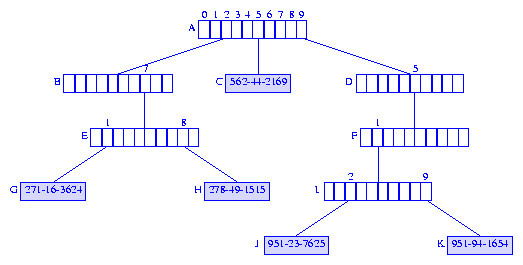Figure 2 Trie for the elements of Figure 1

Searching a Trie
To search a trie for an element with a given key, we start at the root and follow a path down the trie until we either fall off the trie (i.e., we follow a `null` pointer in a branch node) or we reach an element node. The path we follow is determined by the digits of the search key. Consider the trie of Figure 2. Suppose we are to search for an element with key `951-23-7625`. We use the first digit, `9`, in the key to move from the root node `A` to the node `A.child = D`. Since `D` is a branch node, we use the next digit, `5`, of the key to move further down the trie. The node we reach is `D.child = F`. To move to the next level of the trie, we use the next digit, `1`, of the key. This move gets us to the node `F.child = I`. Once again, we are at a branch node and must move further down the trie. For this move, we use the next digit, `2`, of the key, and we reach the element node `I.child = J`. When an element node is reached, we compare the search key and the key of the element in the reached element node. Performing this comparison at node `J`, we get a match. The element in node `J`, is to be returned as the result of the search.

When searching the trie of Figure 2 for an element with key `951-23-1669`, we follow the same path as followed for the key `951-23-7625`. The key comparison made at node `J` tells us that the trie has no element with key `951-23-1669`, and the search returns the value `null`.

To search for the element with key `562-44-2169`, we begin at the root `A` and use the first digit, `5`, of the search key to reach the element node ```A.child = C```. The key of the element in node `C` is compared with the search key. Since the two keys agree, the element in node `C` is returned.

When searching for an element with key `273-11-1341`, we follow the path `A, A.child = B, B.child = E, E.child = null`. Since we fall off the trie, we know that the trie contains no element whose key is `273-11-1341`.

When analyzing the complexity of trie operations, we make the assumption that we can obtain the next digit of a key in `O(1)` time. Under this assumption, we can search a trie for an element with a `d` digit key in `O(d)` time.

Keys With Different Length
In the example of Figure 2, all keys have the same number of digits (i.e., `9`). In applications in which different keys may have a different number of digits, we normally add a special digit (say `#`) at the end of each key so that no key is a prefix of another. To see why this is done, consider the example of Figure 2. Suppose we are to search for an element with the key `27`. Using the search strategy just described, we reach the branch node `E`. What do we do now? There is no next digit in the search key that can be used to reach the terminating condition (i.e., you either fall off the trie or reach an element node) for downward moves. To resolve this problem, we add the special digit `#` at the end of each key and also increase the number of children fields in an element node by one. The additional child field is used when the next digit equals `#`.

Height of a Trie
In the worst case, a root-node to element-node path has a branch node for every digit in a key. Therefore, the height of a trie is at most ```number of digits + 1```.

A trie for social security numbers has a height that is at most `10`. If we assume that it takes the same time to move down one level of a trie as it does to move down one level of a binary search tree, then with at most `10` moves we can search a social-security trie. With this many moves, we can search a binary search tree that has at most `210 - 1 = 1023` elements. This means that, we expect searches in the social security trie to be faster than searches in a binary search tree (for student records) whenever the number of student records is more than `1023`. The breakeven point will actually be less than `1023` because we will normally not be able to construct full or complete binary search trees for our element collection.

Since a SS# is nine digits, a social security trie can have up to `109` elements in it. An AVL tree with `109` elements can have a height that is as much as (approximately) `1.44 log2(109+2) = 44`. Therefore, it could take us four times as much time to search for elements when we organize our student record dictionary as an AVL tree than when this dictionary is organized as a trie!

Space Required and Alternative Node Structures
The use of branch nodes that have as many child fields as the radix of the digits (or one more than this radix when different keys may have different length) results in a fast search algorithm. However, this node structure is often wasteful of space because many of the child fields are `null`. A radix `r` trie for `d` digit keys requires `O(rdn)` child fields, where `n` is the number of elements in the trie. To see this, notice that in a `d` digit trie with `n` information nodes, each information node may have at most `d` ancestors, each of which is a branch node. Therefore, the number of branch nodes is at most `dn`. (Actually, we cannot have this many branch nodes, because the information nodes have common ancestors like the root node.)

We can reduce the space requirements, at the expense of increased search time, by changing the node structure. For example, each branch node of a trie could be replaced by any of the following:
1. A chain of nodes, each node having the three fields `digitValue, child, next`. Node `A` of Figure 2, for example, would be replaced by the chain shown in Figure 3.Figure 3 Chain for node A of Figure 2

The space required by a branch node changes from that required for `r` children/pointer/reference fields to that required for `2p` pointer fields and `p` digit value fields, where `p` is the number of children fields in the branch node that are not `null`. Under the assumption that pointer fields and digit value fields are of the same size, a reduction in space is realized when more than two-thirds of the children fields in branch nodes are `null`. In the worst case, almost all the branch nodes have only `1` field that is not `null` and the space savings become almost `(1 - 3/r) * 100%`.

2. A (balanced) binary search tree in which each node has a digit value and a pointer to the subtrie for that digit value. Figure 4 shows the binary search tree for node `A` of Figure 2.Figure 4 Binary search tree for node A of Figure 2

Under the assumption that digit values and pointers take the same amount of space, the binary search tree representation requires space for `4p` fields per branch node, because each search tree node has fields for a digit value, a subtrie pointer, a left child pointer, and a right child pointer. The binary search tree representation of a branch node saves us space when more than three-fourths of the children fields in branch nodes are `null`. Note that for large `r`, the binary serach tree is faster to search than the chain described above.

3. A binary trie (i.e., a trie with radix `2`). Figure 5 shows the binary trie for node `A` of Figure 2. The space required by a branch node represented as a binary trie is at most `(2 * ceil(log2r) + 1)p`.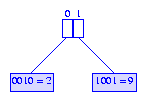Figure 5 Binary trie for node A of Figure 2

4. A hash table. When a hash table with a sufficiently small loading density is used, the expected time performance is about the same as when the node structure of Figure 1 is used. Since we expect the fraction of `null` child fields in a branch node to vary from node to node and also to increase as we go down the trie, maximum space efficiency is obtained by consolidating all of the branch nodes into a single hash table. To accomplish this, each node in the trie is assigned a number, and each parent to child pointer is replaced by a triple of the form ```(currentNode, digitValue, childNode)```. The numbering scheme for nodes is chosen so as to easily distinguish between branch and information nodes. For example, if we expect to have at most `100` elements in the trie at any time, the numbers `0` through `99` are reserved for information nodes and the numbers `100` on up are used for branch nodes. The information nodes are themselves represented as an array `information`. (An alternative scheme is to represent pointers as tuples of the form ```(currentNode, digitValue, childNode, childNodeIsBranchNode)```, where `childNodeIsBranchNode = true` iff the child node is a branch node.)

Suppose that the nodes of the trie of Figure 2 are assigned numbers as given below. This number assignment assumes that the trie will have no more than `10` elements.

```Node      A   B   C   D   E   F   G   H   I   J   K
Number   10  11   0  12  13  14   1   2  15   3   4
```

The pointers in node `A` are represented by the tuples `(10,2,11), (10,5,0)`, and `(10,9,12)`. The pointers in node `E` are represented by the tuples `(13,1,1)` and `(13,8,2)`.

The pointer triples are stored in a hash table using the first two fields (i.e., the `currentNode` and `digitValue`) as the key. For this purpose, we may transform the two field key into an integer using the formula `currentNode * r + digitValue`, where `r` is the trie radix, and use the division method to hash the transformed key into a home bucket. The data presently in information node `i` is stored in `information[i]`.

To see how all this works, suppose we have set up the trie of Figure 2 using the hash table scheme just described. Consider searching for an element with key `278-49-1515`. We begin with the knowledge that the root node is assigned the number `10`. Since the first digit of the search key is `2`, we query our hash table for a pointer triple with key `(10,2)`. The hash table search is successful and the triple `(10,2,11)` is retrieved. The `childNode` component of this triple is `11`, and since all information nodes have a number `9` or less, the child node is determined to be a branch node. We make a move to the branch node `11`. To move to the next level of the trie, we use the second digit `7` of the search key. For the move, we query the hash table for a pointer with key `(11,7)`. Once again, the search is successful and the triple `(11,7,13)` is retrieved. The next query to the hash table is for a triple with key `(13,8)`. This time, we obtain the triple `(13,8,2)`. Since, ```childNode = 2 < 10```, we know that the pointer gets us to an information node. So, we compare the search key with the key of the element `information`. The keys match, and we have found the element we were looking for.

When searching for an element with key `322-167-8976`, the first query is for a triple with key `(10,3)`. The hash table has no triple with this key, and we conclude that the trie has no element whose key equals the search key.

The space needed for each pointer triple is about the same as that needed for each node in the chain of nodes representation of a trie node. Therefore, if we use a linear open addressed hash table with a loading density of `alpha`, the hash table scheme will take approximately `(1/alpha - 1) * 100%` more space than required by the chain of nodes scheme. However, when the hash table scheme is used, we can retrieve a pointer in `O(1)` expected time, whereas the time to retrieve a pointer using the chain of nodes scheme is `O(r)`. When the (balanced) binary search tree or binary trie schemes are used, it takes `O(log r)` time to retrieve a pointer. For large radixes, the hash table scheme provides significant space saving over the scheme of Figure 2 and results in a small constant factor degradation in the expected time required to perform a search.

The hash table scheme actually reduces the expected time to insert elements into a trie, because when the node structure of Figure 2 is used, we must spend `O(r)` time to initialize each new branch node (see the description of the insert operation below). However, when a hash table is used, the insertion time is independent of the trie radix.

To support the removal of elements from a trie represented as a hash table, we must be able to reuse information nodes. This reuse is accomplished by setting up an available space list of information nodes that are currently not in use (see Section 3.5 (Simulating Pointers) of the text).

Inserting into a Trie
To insert an element `theElement` whose key is `theKey`, we first search the trie for an existing element with this key. If the trie contains such an element, then we replace the existing element with `theElement`. When the trie contains no element whose key equals `theKey`, `theElement` is inserted into the trie using the following procedure.

Case 1 For Insert Procedure
If the search for `theKey` ended at an element node `X`, then the key of the element in `X` and `theKey` are used to construct a subtrie to replace `X`.

Suppose we are to insert an element with key `271-10-2529` into the trie of Figure 2. The search for the key `271-10-2529` terminates at node `G` and we determine that the key, `271-16-3624`, of the element in node `G` is not equal to the key of the element to be inserted. Since the first three digits of the keys are used to get as far as node `E` of the trie, we set up branch nodes for the fourth digit (from the left) onwards until we reach the first digit at which the two keys differ. This results in branch nodes for the fourth and fifth digits followed by element nodes for each of the two elements. Figure 6 shows the resulting trie.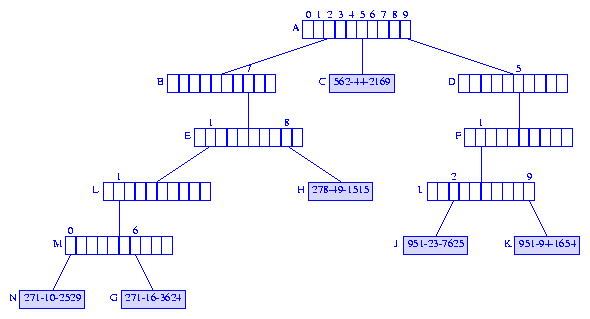Figure 6 Trie of Figure 2 with 271-10-2529 inserted

Case 2 For Insert Procedure
If the search for `theKey` ends by falling off the trie from the branch node `X`, then we simply add a child (which is an element node) to the node `X`. The added element node contains `theElement`.

Suppose we are to insert an element with key `987-33-1122` to the trie of Figure 2. The search for an element with key equal to `987-33-1122` ends when we fall off the trie while following the pointer `D.child`. We replace the `null` pointer `D.child` with a pointer to a new element node that contains `theElement`, as is shown in Figure 7.Figure 7 Trie of Figure 2 with 987-33-1122 inserted

The time required to insert an element with a `d` digit key into a radix `r` trie is `O(dr)` because the insertion may require us to create `O(d)` branch nodes and it takes `O(r)` time to intilize the children pointers in a branch node.

Removing an Element
To remove the element whose key is `theKey`, we first search for the element with this key. If there is no matching element in the trie, nothing is to be done. So, assume that the trie contains an element `theElement` whose key is `theKey`. The element node `X` that contains `theElement` is discarded, and we retrace the path from `X` to the root discarding branch nodes that are roots of subtries that have only `1` element in them. This path retracing stops when we either reach a branch node that is not discarded or we discard the root.

Consider the trie of Figure 7. When the element with key `951-23-7625` is removed, the element node `J` is discarded and we follow the path from node `J` to the root node `A`. The branch node `I` is discarded because the subtrie with root `I` contains the single element node `K`. We next reach the branch node `F`. This node is also discarded, and we proceed to the branch node `D`. Since the subtrie rooted at `D` has `2` element nodes (`K` and `L`), this branch node is not discarded. Instead, node `K` is made a child of this branch node, as is shown in Figure 8.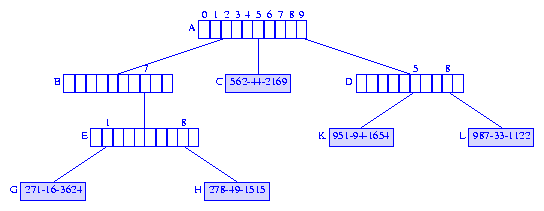Figure 8 Trie of Figure 7 with 951-23-7635 removed

To remove the element with key `562-44-2169` from the trie of Figure 8, we discard the element node `C`. Since its parent node remains the root of a subtrie that has more than one element, the parent node is not discarded and the removal operation is complete. Figure 9 show the resulting trie.Figure 9 Trie of Figure 8 with 562-44-2169 removed

The time required to remove an element with a `d` digit key from a radix `r` trie is `O(dr)` because the removal may require us to discard `O(d)` branch nodes and it takes `O(r)` time to determine whether a branch node is to be discarded. The complexity of the remove operation can be reduced to `O(r+d)` by adding a `numberOfElementsInSubtrie` field to each branch node.

Prefix Search and Applications
You have probably realized that to search a trie we do not need the entire key. Most of the time, only the first few digits (i.e., a prefix) of the key is needed. For example, our search of the trie of Figure 2 for an element with key `951-23-7625` used only the first four digits of the key. The ability to search a trie using only the prefix of a key enables us to use tries in applications where only the prefix might be known or where we might desire the user to provide only a prefix. Some of these applications are described below.

Criminology
Suppose that you are at the scene of a crime and observe the first few characters `CRX` on the registration plate of the getaway car. If we have a trie of registration numbers, we can use the characters `CRX` to reach a subtrie that contains all registration numbers that begin with `CRX`. The elements in this subtrie can then be examined to see which cars satisfy other properties that might have been observed.

Automatic Command Completion
When using an operating system such as Unix or DOS, we type in system commands to accomplish certain tasks. For example, the Unix and DOS command `cd` may be used to change the current directory. Figure 10 gives a list of commands that have the prefix `ps` (this list was obtained by executing the command `ls /usr/local/bin/ps*` on a Unix system).

```ps2ascii      ps2pdf      psbook         psmandup      psselect
ps2epsi       ps2pk       pscal          psmerge       pstopnm
ps2frag       ps2ps       psidtopgm      psnup         pstops
ps2gif        psbb        pslatex        psresize      pstruct
```
Figure 10 Commands that begin with "ps"

We can simply the task of typing in commands by providing a command completion facility which automatically types in the command suffix once the user has typed in a long enough prefix to uniquely identify the command. For instance, once the letters `psi` have been entered, we know that the command must be `psidtopgm` because there is only one command that has the prefix `psi`. In this case, we replace the need to type in a `9` character command name by the need to type in just the first `3` characters of the command!

A command completion system is easily implemented when the commands are stored in a trie using ASCII characters as the digits. As the user types the command digits from left to right, we move down the trie. The command may be completed as soon as we reach an element node. If we fall off the trie in the process, the user can be informed that no command with the typed prefix exists.

Although we have described command completion in the context of operating system commands, the facilty is useful in other environments:
1. A network browser keeps a history of the URLs of sites that you have visited. By organizing this history as a trie, the user need only type the prefix of a previously used URL and the browser can complete the URL.
2. A word processor can maintain a dictionary of words and can complete words as you type the text. Words can be completed as soon as you have typed a long enough prefix to identify the word uniquely.
3. An automatic phone dialler can maintain a list of frequently called telephone numbers as a trie. Once you have punched in a long enough prefix to uniquely identify the phone number, the dialler can complete the call for you.

LZW Compression Algorithm
The LZW compression algorithm was developed in Section 7.5 of the text. Recall that the primary operation performed during compression is the determination of the longest prefix (of the uncompressed portion of the file that is begin compressed) for which we have a code in the code table. Let us call this operation `longestPrefix`.

In Section 7.5.2, the code table was implemented as a hash table and the operation `longestPrefix` was performed by searching this hash table first using a prefix of size `1`, then a prefix of size `2`, and so on until the first unsuccessful search. Since each hash table search has an expected complexity of `O(1)`, a `longestPrefix` operation takes `O(s)` expected time, where `s` is the length of the longest prefix for which we have a code in our code table.

We may use a trie for the code table. In this application, we add a `code` field to each branch node. The `code` field of a branch node gives the code for the string defined by the path from the trie root to this branch node. The trie for LZW codes has no element nodes.

The `longestPrefix` operation may be implemented as follows. Scan the characters in the uncompressed portion of the file from left to right. Use these characters to follow a path down the code trie until you fall off the trie. Once you fall off, the longest matching prefix is identified, and the code for this prefix is in the `code` field of the branch node you fell off of.

Using a trie, the actual complexity of the `longestPrefix` operation is `O(s)`.

Compressed Tries
Take a close look at the trie of Figure 2. This trie has a few branch nodes (nodes `B, D,` and `F`) that do not partition the elements in their subtrie into two or more nonempty groups. We often can improve both the time and space performance metrics of a trie by eliminating all branch nodes that have only one child. The resulting trie is called a compressed trie.

When branch nodes with a single child are removed from a trie, we need to keep additional information so that dictionary operations may be performed correctly. The additional information stored in three compressed trie structures is described below.

Compressed Tries with Digit Numbers
In a compressed trie with digit numbers, each branch node has an additional field `digitNumber` that tells us which digit of the key is used to branch at this node. Figure 11 shows the compressed trie with digit numbers that corresponds to the trie of Figure 2. The leftmost field of each branch node of Figure 11 is the `digitNumber` field.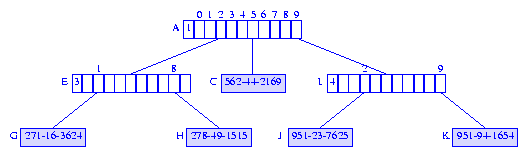Figure 11 Compressed trie with digit numbers

Searching a Compressed Trie with Digit Numbers
A compressed trie with digit numbers may be searched by following a path from the root. At each branch node, the digit, of the search key, given in the branch node's `digitNumber` field is used to determine which subtrie to move to. For example, when searching the trie of Figure 11 for an element with key `951-23-7625`, we start at the root of the trie. Since the root node is a branch node with `digitNumber = 1`, we use the first digit `9` of the search key to determine which subtrie to move to. A move to node ```A.child = I is made. Since, I.digitNumber = 4, the fourth digit, 2, of the search key tells us which subtrie to move to. A move is now made to node I.child = J. We are now at an element node, and the search key is compared with the key of the element in node J. Since the keys match, we have found the desired element. Notice that a search for an element with key 913-23-7625 also terminates at node J. However, the search key and the element key at node J do not match and we conclude that the trie contains no element with key 913-23-7625. Inserting into a Compressed Trie with Digit Numbers To insert an element with key 987-26-1615 into the trie of Figure 11, we first search for an element with this key. The search ends at node J. Since, the search key and the key, 951-23-7625, of the element in this node do not match, we conclude that the trie has no element whose key matches the search key. To insert the new element, we find the first digit where the search key differs from the key in node J and create a branch node for this digit. Since, the first digit where the search key 987-26-1615 and the element key 951-23-7625 differ is the second digit, we create a branch node with digitNumber = 2. Since, digit values increase as we go down the trie, the proper place to insert the new branch node can be determined by retracing the path from the root to node J and stopping as soon as either a node with digit value greater than 2 or the node J is reached. In the trie of Figure 11, this path retracing stops at node I. The new branch node is made the parent of node I, and we get the trie of Figure 12.Figure 12 Compressed trie following the insertion of 987-26-1615 into the compressed trie of Figure 11 Consider inserting an element with key 958-36-4194 into the compressed trie of Figure 11. The search for an element with this key terminates when we fall of the trie by following the pointer I.child = null. To complete the insertion, we must first find an element in the subtrie rooted at node I. This element is found by following a downward path from node I using (say) the first non null link in each branch node encountered. Doing this on the compressed trie of Figure 11, leads us to node J. Having reached an element node, we find the first digit where the element key and the search key differ and complete the insertion as in the previous example. Figure 13 shows the resulting compressed trie.Figure 13 Compressed trie following the insertion of 958-36-4194 into the compressed trie of Figure 11 Because of the possible need to search for the first non null child pointer in each branch node, the time required to insert an element into a compressed tries with digit numbers is O(rd), where r is the trie radix and d is the maximum number of digits in any key. Removing an Element from a Compressed Trie with Digit Numbers To remove an element whose key is theKey, we do the following: Find the element node X that contains the element whose key is theKey. Discard node X. If the parent of X is left with only one child, discard the parent node also. When the parent of X is discarded, the sole remaining child of the parent of X becomes a child of the grandparent (if any) of X. To remove the element with key 951-94-1654 from the compressed trie of Figure 13, we first locate the node K that contains the element that is to be removed. When this node is discarded, the parent I of K is left with only one child. Consequently, node I is also discarded, and the only remaining child J of node I is the made a child of the grandparent of K. Figure 14 shows the resulting compressed trie.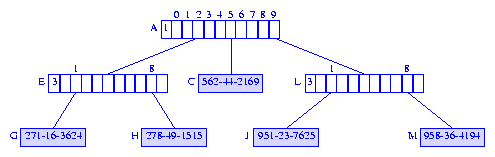Figure 14 Compressed trie following the removal of 951-94-1654 from the compressed trie of Figure 13 Because of the need to determine whether a branch node is left with two or more children, removing a d digit element from a radix r trie takes O(d+r) time. Compressed Tries with Skip Fields In a compressed trie with skip fields, each branch node has an additional field skip which tells us the number of branch nodes that were originally between the current branch node and its parent. Figure 15 shows the compressed trie with skip fields that corresponds to the trie of Figure 2. The leftmost field of each branch node of Figure 15 is the skip field.Figure 15 Compressed trie with skip fields The algorithms to search, insert, and remove are very similar to those used for a compressed trie with digit numbers. Compressed Tries with Edge Information In a compressed trie with edge information, each branch node has the following additional information associated with it: a pointer/reference element to an element (or element node) in the subtrie, and an integer skip which equals the number of branch nodes eliminated between this branch node and its parent. Figure 16 shows the compressed trie with edge information that corresponds to the trie of Figure 2. The first field of each branch node is its element field, and the second field is the skip field.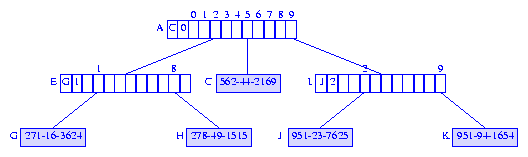Figure 16 Compressed trie with edge information Even though we store the ``edge information'' with branch nodes, it is convenient to think of this information as being associated with the edge that comes into the branch node from its parent (when the branch node is not the root). When moving down a trie, we follow edges, and when an edge is followed. we skip over the number of digits given by the skip field of the edge information. The value of the digits that are skipped over may be determined by using the element field. When moving from node A to node I of the compressed trie of Figure 16, we use digit 1 of the key to determine which child field of A is to be used. Also, we skip over the next 2 digits, that is, digits 2 and 3, of the keys of the elements in the subtrie rooted at I. Since all elements in the subtrie I have the same value for the digits that are skipped over, we can determine the value of these skipped over digits from any of the elements in the subtrie. Using the element field of the edge information, we access the element node J, and determine that the digits that are skipped over are 5 and 1. Searching a Compressed Trie with Edge Information When searching a compressed trie with edge information, we can use the edge information to terminate unsuccessful searches (possibly) before we reach an element node or fall off the trie. As in the other compressed trie variants, the search is done by following a path from the root. Suppose we are searching the compressed trie of Figure 16 for an element with key 921-23-1234. Since the skip value for the root node is 0, we use the first digit 9 of the search key to determine which subtrie to move to. A move to node A.child = I is made. By examining the edge information (stored in node I), we determine that, in making the move from node A to node I, the digits 5 and 1 are skipped. Since these digits do not agree with the next two digits of the search key, the search terminates with the conclusion that the trie contains no element whose key equals the search key. Inserting into a Compressed Trie with Edge Information To insert an element with key 987-26-1615 into the compressed trie of Figure 16, we first search for an element with this key. The search terminates unsuccessfully when we move from node A to node I because of a mismatch between the skipped over digits and the corresponding digits of the search key. The first mismatch is at the first skipped over digit. Therefore, we insert a branch node L between nodes A and I. The skip value for this branch node is 0, and its element field is set to reference the element node for the newly inserted element. We must also change the skip value of I to 1. Figure 17 shows the resulting compressed trie.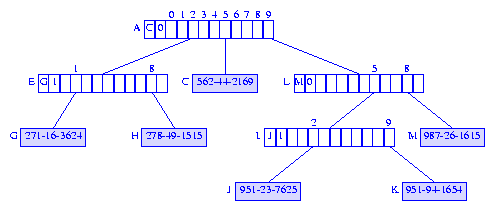Figure 17 Compressed trie following the insertion of 987-26-1615 into the compressed trie of Figure 16 Suppose we are to insert an element with key 958-36-4194 into the compressed trie of Figure 16. The search for an element with this key terminates when we move to node I because of a mismatch between the digits that are skipped over and the corresponding digits of the search key. A new branch node is inserted between nodes A and I and we get the compressed trie that is shown in Figure 18.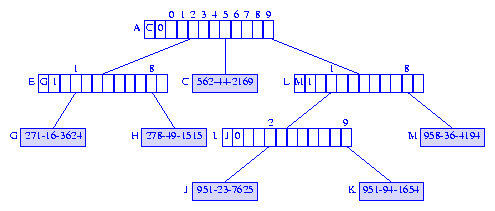Figure 18 Compressed trie following the insertion of 958-36-4194 into the compressed trie of Figure 16 The time required to insert a d digit element into a radix r compressed trie with edge information is O(r+d). Removing an Element from a Compressed Trie with Edge Information This is similar to removal from a compressed trie with digit numbers except for the need to update the element fields of branch nodes whose element field references the removed element. Space Required by a Compressed Trie Since each branch node partitions the elements in its subtrie into two or more nonempty groups, an n element compressed trie has at most n-1 branch nodes. Therefore, the space required by each of the compressed trie variants described by us is O(nr), where r is the trie radix. When compressed tries are represented as hash tables, we need an additional data structure to store the nonpointer fields of branch nodes. We may use an array (much like we use the array information) for this purpose. Exercises ```
``` Draw the compressed trie with digit numbers for the keys aabbbbaaa, bbbaaabb, aaabaabb, aaaaabaaa, bbbaabaa, and aabba. You will need to append a special symbol (code class=var># to each of the keys. ```
1. ``` Draw the information on edge compressed trie for the keys aabbbbaaa, bbbaaabb, aaabaabb, aaaaabaaa, bbbaabaa, and aabba. You will need to append a special symbol (code class=var>#``` to each of the keys.
2. Develop the class `CompressedTrieWithDigitNumber` which implements compressed tries with digit numbers. Use nodes that have as many children fields as the alphabet size plus one (for the special symol at the end of each key). You must include methods to search (both exact match and prefix match), insert, and remove.
3. Develop the class `CompressedTrieWithDigitNumber` which implements compressed tries with digit numbers. Use the hash table scheme. You must include methods to search (both exact match and prefix match), insert, and remove.
4. Develop the class `CompressedTrieWithEdgeInformation` which implements compressed tries with digit numbers. Use nodes that have as many children fields as the alphabet size plus one (for the special symol at the end of each key). You must include methods to search (both exact match and prefix match), insert, and remove.
5. Develop the class `CompressedTrieWithEdgeInformation` which implements compressed tries with digit numbers. Use the hash table scheme. You must include methods to search (both exact match and prefix match), insert, and remove.